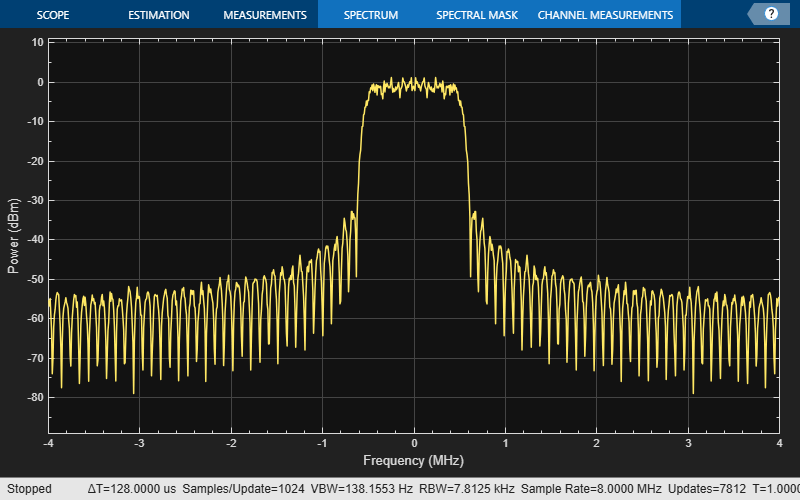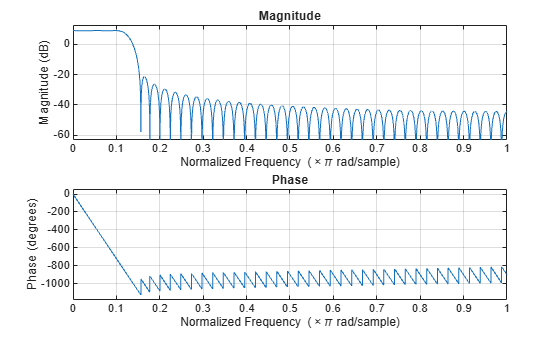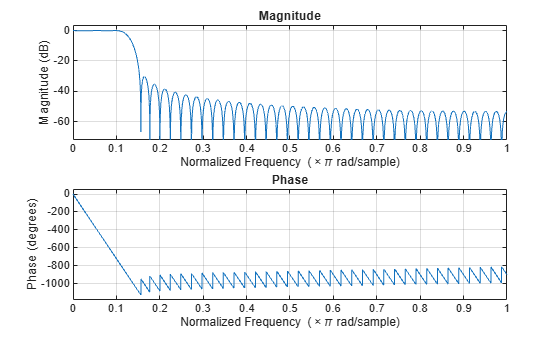# comm.RaisedCosineTransmitFilter

Apply pulse shaping by interpolating signal using raised-cosine FIR filter

## Description

The `comm.RaisedCosineTransmitFilter` System object™ applies pulse shaping by interpolating an input signal using a raised cosine finite impulse response (FIR) filter. The FIR filter has (`FilterSpanInSymbols` × `OutputSamplesPerSymbol` + 1) tap coefficients.

To apply pulse shaping by interpolating an input signal using a raised cosine FIR filter:

1. Create the `comm.RaisedCosineTransmitFilter` object and set its properties.

2. Call the object with arguments, as if it were a function.

## Creation

### Syntax

``txfilter = comm.RaisedCosineTransmitFilter``
``txfilter = comm.RaisedCosineTransmitFilter(Name,Value)``

### Description

````txfilter = comm.RaisedCosineTransmitFilter` returns a raised cosine transmit FIR filter System object, which interpolates an input signal using a raised cosine FIR filter. The filter uses an efficient polyphase FIR interpolation structure and has unit energy.```

example

````txfilter = comm.RaisedCosineTransmitFilter(Name,Value)` sets properties using one or more name-value pairs. Enclose each property name in quotes. For example, `comm.RaisedCosineTransmitFilter('FilterSpanInSymbols',15)` configures a raised cosine transmit filter System object with the filter span set to 15 symbols.```

## Properties

expand all

Unless otherwise indicated, properties are nontunable, which means you cannot change their values after calling the object. Objects lock when you call them, and the `release` function unlocks them.

If a property is tunable, you can change its value at any time.

Filter shape, specified as `'Square root'` or `'Normal'`.

Data Types: `char` | `string`

Roll-off factor, specified as a scalar in the range [0, 1].

Data Types: `double`

Filter span in symbols, specified as a positive integer. The object truncates the infinite impulse response (IIR) of an ideal raised-cosine filter to an impulse response that spans the number of symbols specified by this property.

Data Types: `double`

Output samples per symbol, specified as a positive integer.

Data Types: `double`

Linear filter gain, specified as a positive scalar. The object designs a raised-cosine filter that has unit energy and then applies the linear filter gain to obtain final tap coefficient values.

Data Types: `double`

## Usage

### Syntax

``y = txfilter(x)``

### Description

example

``` `y = txfilter(x)` applies pulse shaping by interpolating an input signal using a raised cosine FIR filter. The output consists of interpolated signal values.```

### Input Arguments

expand all

Input signal, specified as a column vector or a Ki-by-N matrix. Ki is the number of input samples per signal channel, and N is the number of signal channels.

For a Ki-by-N matrix input, the object processes columns of the input matrix as N independent channels.

Data Types: `double` | `single`
Complex Number Support: Yes

### Output Arguments

expand all

Output signal, returned as a column vector or a Ko-by-N matrix. Ko is equal to Ki × `OutputSamplesPerSymbol`. Ki is the number of input samples per signal channel, and N is the number of signal channels.

The object interpolates and filters each channel over the first dimension and then generates a Ko-by-N output matrix. The output signal is the same data type as the input signal.

## Object Functions

To use an object function, specify the System object as the first input argument. For example, to release system resources of a System object named `obj`, use this syntax:

`release(obj)`

expand all

 `info` Information about filter System object `coeffs` Coefficients for filters `cost` Computational cost of implementing filter System object `freqz` Frequency response of discrete-time filter `fvtool` Plot frequency response of filter `grpdelay` Group delay response of discrete-time filter `impz` Impulse response of discrete-time filter `order` Order of discrete-time filter System object
 `step` Run System object algorithm `release` Release resources and allow changes to System object property values and input characteristics `reset` Reset internal states of System object

## Examples

collapse all

Interpolate a signal using square-root-raised-cosine (SRRC) transmit filter object and display the spectrum of the filtered signal.

Create random bipolar symbols at a symbol rate of 1e6 symbols per second.

`data = 2*randi([0 1],1e6,1) - 1;`

Create a SRRC transmit filter object. The default sets the filter to a square-root shape and the number of samples per symbol to 8.

`txfilter = comm.RaisedCosineTransmitFilter`
```txfilter = comm.RaisedCosineTransmitFilter with properties: Shape: 'Square root' RolloffFactor: 0.2000 FilterSpanInSymbols: 10 OutputSamplesPerSymbol: 8 Gain: 1 ```

Filter the data by using the SRRC filter.

`filteredData = txfilter(data);`

Create a spectrum analyzer object with an 8e6 sampling rate. This sampling rate matches the sampling rate of the filtered signal.

`spectrumAnalyzer = dsp.SpectrumAnalyzer('SampleRate',8e6);`

View the spectrum of the filtered signal by using the spectrum analyzer object.

`spectrumAnalyzer(filteredData)`Create interpolated signals from a square-root-raised-cosine (SRRC) filter with various filter spans. Examine the magnitude response of the various filter designs.

Create SRRC filter objects setting various filter spans. Use the `coeffs` object function to obtain the filter coefficients.

```txfilt2 = comm.RaisedCosineTransmitFilter('FilterSpanInSymbols',2); txfilt4 = comm.RaisedCosineTransmitFilter('FilterSpanInSymbols',4); txfilt6 = comm.RaisedCosineTransmitFilter('FilterSpanInSymbols',6); txfilt8 = comm.RaisedCosineTransmitFilter('FilterSpanInSymbols',8); txfilt16 = comm.RaisedCosineTransmitFilter('FilterSpanInSymbols',16); taps2 = coeffs(txfilt2).Numerator; taps4 = coeffs(txfilt4).Numerator; taps6 = coeffs(txfilt6).Numerator; taps8 = coeffs(txfilt8).Numerator; taps16 = coeffs(txfilt16).Numerator;```

Launch the filter visualization tool to show the magnitude response for various filter spans. Specify a sample rate of 1 kHz. Display the two-sided centered response.

```fvt = fvtool(taps2,1,taps4,1,taps8,1,taps16,1); fvt.Fs = 1e3; fvt.FrequencyRange = '[-Fs/2, Fs/2)'; legend(fvt,'Span 2 symbols','Span 4 symbols', ... 'Span 8 symbols','Span 10 symbols')```Create a square-root-raised-cosine (SRRC) transmit filter System object™, and then plot the filter response. The results show that the linear filter gain is greater than unity. Specifically, the passband gain is greater than 0 dB.

```txfilter = comm.RaisedCosineTransmitFilter; fvtool(txfilter)```Obtain the filter coefficients by using the `coeffs` object function and adjust the filter gain to unit energy.

`b = coeffs(txfilter);`

Because a filter with unity passband gain must have filter coefficients that sum to 1, set the linear filter gain to the inverse of the sum of the filter tap coefficients, `b.Numerator`.

`txfilter.Gain = 1/sum(b.Numerator);`

Verify that the resulting filter coefficients sum to 1.

```bNorm = coeffs(txfilter); sum(bNorm.Numerator)```
```ans = 1.0000 ```

Plot the filter frequency response again. The results now show that the passband gain is 0 dB, which is unity gain.

`fvtool(txfilter)`## Version History

Introduced in R2013b